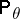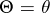# A priori distribution

(diff) ← Older revision | Latest revision (diff) | Newer revision → (diff)
The probability distribution of a random variable, to be contrasted with the conditional distribution of this random variable under certain additional conditions. Usually the term "a priori distribution" is used in the following way. Letbe a pair of random variables (random vectors or more general random elements). The random variableis considered to be unknown, whileis considered to be the result of an observation to be used for estimation of. The joint distribution ofandis given by the distribution of(now called the a priori distribution) and the set of conditional probabilitiesof the random variablegiven. According to the Bayes formula, one can calculate the conditional probability ofwith respect to(which is now called the a posteriori distribution of). In statistical problems, the a priori distribution is often unknown (and even the assumption on its existence is not sufficiently founded). For the use of the a priori distribution, see Bayesian approach.